# Appendix C: Present and Future Value Concepts

Business

Compare options using present value concepts: (1) In this option winner receives \$1 million per year for next 20 years. Therefore, let us use present value of annuity table C-4 to calculate the value of future receivables today. Let us look into table C-4 for 10% column and 20 period's row to find the factors 8.5136.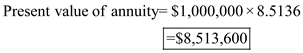(2) In this option the winner receives \$8,000,000 today. Now the present value is \$8,000,000 only. (3) This option involves two receipts of \$2,000,000 today and \$700,000 for each year for the next 20 years. Now let us add these two receipts to determine the present value of this option. The present value of \$2,000,000 today is \$2,000,000 only. Receipt of \$700,000 for each year for the next 20 years is calculated as follows.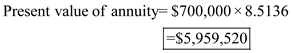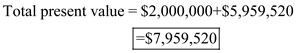First option yields \$8,513,600 second option yields \$8,000,000 and third option yields \$7,959,520. As first option produces more income, it is preferable to select the same. Generally, more income option would be selected as it influences one's decision.

(1) Compute the balance in the savings account at the end of 10 years: In order to calculate the present value of an annuity for \$6,000 each year in savings account at 10% at annual compound interest for 10 periods, let us look into table C-3 at 10% column and 10 years row.(2) Compute the interest for the 10 years:(3) Compute the interest revenue for 2013 and 2014: For 2013: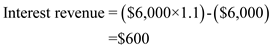For 2014: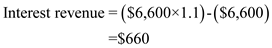To know the future value of \$15,000 deposit for next 10 years to earn 5% each year we have to consider table C.3- future value of annuity of \$1. Let us calculate the future value of annuity of deposit of \$15,000 of each period is as follows.The correct option is (b). Therefore, all the remaining options (a), (c), and (d) are incorrect as they show incorrect value of dollar amounts.